Study Materials

# NCERT Solutions for Class 9th Mathematics

Page 4 of 5

## Chapter 2. Polynomials

### Exercise 2.4

Chapter 2. Polynomials

Exercise 2.4

Q.1. Determine which of the following polynomials has (x+ 1) a factor:

(i) x+x+ x +1                                        (ii) x+ x+ x+ x +1

(iii) x+ 3x+ 3x+ x +1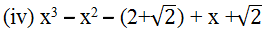Solution:

(i) If (x + 1) is a factor of p(x) = x3+ x2+ x + 1, then p (−1) must be zero, otherwise (x + 1) is not a factor of p(x).

P(x)  = x+ x+ x + 1

P(−1)= (−1)+ (−1)+ (−1) + 1

= − 1 + 1 − 1 − 1

= 0

∵ P(x) = 0

Hence, x + 1 is a factor of this polynomial.

(ii) If (x + 1) is a factor of p(x) = x4+ x3+ x2+ x + 1, then p (−1) must be zero, Otherwise (x + 1) is not a factor of p(x).

P(x) = x4+ x3+ x2+ x + 1

P(−1) = (−1)+ (−1)+ (−1)+ (−1) + 1

= 1 − 1 + 1 −1 + 1

= 1

As P(x) ≠ 0, (− 1)

Therefore, x + 1 is not a factor of this polynomial.

(iii) If (x + 1) is a factor of polynomial p(x) = x4+ 3x3+ 3x2+ x + 1, then p (−1) must  be 0, otherwise (x + 1) is not a factor of this polynomial.

P(x) = x4+ 3x3+ 3x2+ x + 1

P(−1) = (−1)4+ 3(−1)3+ 3(−1)2+ (−1) + 1

= 1 − 3 + 3 − 1 + 1

= 1

As P(x) ≠ 0, (−1)

Therefore (x+1) is not a factor of this polynomial .Q.2. Use the Factor Theorem to determine whether g(x) is a factor of p(x) in each of the following cases:

(i)         P(x) = 2x3+ x2− 2x − 1, g(x) = x + 1

(ii)        P(x) = x+ 3x+ 3x + 1, g(x) = x + 2

(iii)       P(x) = x− 4 x+  x + 6, g(x) = x − 3

Solution:

(i)   If g(x) = x + 1 is a factor of the given polynomial p(x), then p (−1) must be zero.

P (x) = 2x+ x− 2x − 1

P (−1) = 2(−1)3+ (−1)2− 2(−1) − 1

= 2(−1) + 1 + 2 – 1

= 0

∵ P(x) = 0

Hence, g(x) = x + 1 is a factor of the given polynomial.

(ii) If g(x) = x + 2 is a factor of the given polynomial p(x), then p (−2) must be 0.

P (x) = x3+3x2+ 3x + 1

P (−2) = (−2)3+ 3(−2)2+ 3(−2) + 1

= − 8 + 12 − 6 + 1

= −1

As P(x) ≠ 0,

Hence, g(x) = x + 2 is not a factor of the given polynomial.

(iii) If g(x) = x − 3 is a factor of the given polynomial p(x), then P(3) must be 0.

P(x) = x3− 4 x2+ x + 6

P(3) = (3)− 4(3)+ 3 + 6

= 27 −36 + 9

= 0

Hence, g(x) = x − 3 is a factor of the given polynomial.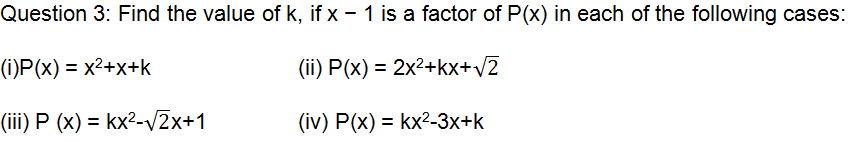Solution:

: If x − 1 is a factor of polynomial p(x), then P(1) must be 0.

(i) P(x) = x2+ x + k

P(1) = (1)2+ 1 + k

= 1+1+

= 2+k

k  =−2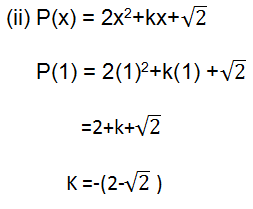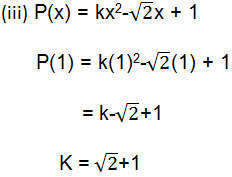(iv) P(x) = kx2-3x + k

P(1) = k(1)2-3(1) + k

= k-3+k

2k = 3

K = 3/2

Question 4: Factorise:

(i) 12x2− 7x + 1                           (ii) 2x2+ 7x + 3

(iii) 6x2+ 5x – 6                           (iv) 3x2− x − 4

Solution:

(i) 12x2− 7x + 1 we can find two numbers,

Such that pq = 12 × 1 = 12 and p + q = −7.

They are p = −4 and q = −3

Here, 12x2− 7x + 1

= 12x2− 4x − 3x + 1

= 4x (3x − 1) − 1 (3x − 1)

= (3x − 1) (4x − 1)

(ii) 2x2+ 7x + 3 we can find two numbers such that pq = 2 × 3= 6 and p + q = 7.

They are p = 6 and q = 1.

Here, 2x2 + 7x + 3

= 2x2+ 6x + x + 3

= 2x (x + 3) + 1 (x + 3)

= (x + 3) (2x+ 1)

(iii) 6x2+ 5x − 6 we can find two numbers such that pq = −36 and p + q = 5.

They are p = 9 and q = −4.

Here, 6x2+ 5x – 6

= 6x2+ 9x − 4x – 6

= 3x (2x + 3) − 2 (2x + 3)

= (2x + 3) (3x − 2)

(iv)  3x2− x − 4 we can find two numbers,

such that pq = 3 × (−4) = −12 and p + q = −1.

They are p = −4 and q = 3

Here, 3x2− x − 4

= 3x2− 4x + 3x – 4

= x (3x − 4) + 1 (3x − 4)

= (3x − 4) (x + 1)

Question 5. Factorize:

(i) x3− 2x2− x + 2                 (ii) x3+ 3x2−9x − 5

(iii) x3+ 13x2+ 32x + 20         (iv) 2y3+ y2− 2y – 1

Solution:

(i) Let P(x) = x3− 2x2− x + 2 all the factor are there. These are ± 1, ± 2.

By trial method, P (1) = (1)3− 2(1)2− 1 + 2

= 1 − 2 − 1+ 2

= 0 Therefore, (x − 1) is factor of polynomial p(x)

Let us find the quotient on dividing x3− 2x2− x + 2 by x − 1.

By long division methodNow,

Dividend = Divisor × Quotient + remainder

x3− 2x2− x + 2 = (x – 1) ( X2– x – 2) + 0

= (x – 1) (x2–2x+x–2)

= (x – 1) [x (x–2) + 1(x–2)]

= (x – 1) (x + 1) (x – 2)

(ii)   Let p(x) = x3 – 3x2−9x – 5 all the factor are there. These are ± 1, ± 2.

By trial method, p (–1) = (–1)3– 3(1)2− 9(1) – 5

= –1– 3–9–5     =0

Therefore (x+1) is the factor of polynomial p(x).

.   Let us find the quotient on dividing x3– 3x2−9x – 5 by x+1.

By long division methodNow,

Dividend = Divisor × Quotient + remainder

x3– 3x2−9x – 5 = (x +1) ( X2–4x – 5) + 0

=(x + 1) (x2–5x+x–5)

=(x + 1) [x (x–5) +1(x–5)]

=(x + 1) (x + 1) (x – 5)

(iii)  Let p(x) = x3+ 13x2+ 32x + 20    all the factor are there.

These are ± 1, ± 2, ± 3, ± 4.

By trial method, p (–1) = (–1)3+13(–1)2+ 32(–1) +20

= –1+13–32+20   = 0

Therefore (x+1) is the factor of polynomial p(x).

Let us find the quotient on dividing x3+ 13x2+ 32x + 20 by x+1

By long division methodNow,

Dividend = Divisor × Quotient + remainder

x3 +13x+ 32x + 20 = (x +1) ( x2 + 12x + 20) + 0

=(x + 1) (x2+10x+2x+20)

=(x + 1) [x (x+10) +2(x+10)]

=(x + 1) (x + 2) (x + 10)

(iv) Let p(y) = 2y3+ y2− 2y – 1 all the factor are there. These are ± 1, ± 2.

By trial method, p (1) =2(1)3 + (1)2 – 2(1) – 1

=2 + 1 – 2 – 1    =0

Therefore (y–1) is the factor of polynomial p(y).

Let us find the quotient on dividing 2y3+ y2− 2y – 1 by y–1.

By long division method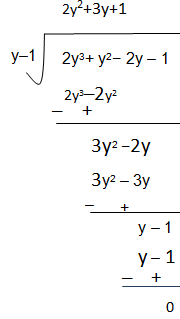Now,

Dividend = Divisor × Quotient + remainder

2y3+ y2− 2y −1 =(y − 1) (2y2+3y + 1)

= (y − 1) (2y2+2y

= (y − 1) [2y (y+1) + 1 (y + 1)]

= (y − 1) (y + 1) (2y + 1)

Page 4 of 5

Chapter Contents: